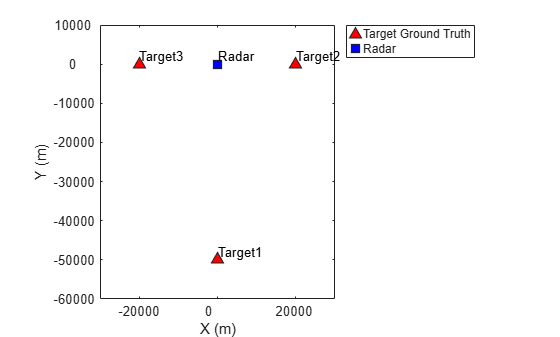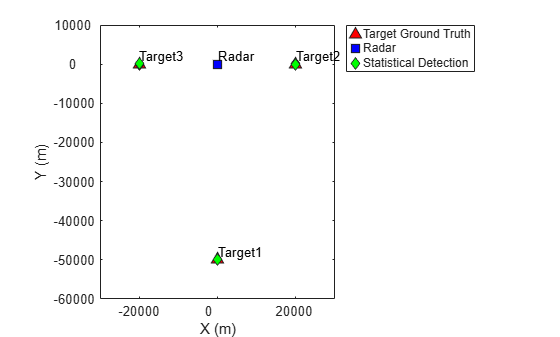# Create Physics-Based Radar Model from Statistical Model

This example shows how to programmatically create a physics-based radar model from a statistical radar model.

A radar is a perception system that uses an antenna or antenna array to capture RF energy, which is then downconverted and processed to provide information on objects in the radar's field of view. The received signal needs to pass through both a signal processing subsystem and a data processing subsystem.

The goal of the signal processing subsystem is to translate received IQ signals to target detections. The data processing subsystem takes those detections and produces tracks corresponding to the detected targets.

The signal processing subsystem helps to generate a snapshot of the scene at the current time and includes information on whether an object is in the coverage and, if so, where it is. The data processing subsystem links those snapshots together so operators can understand what happened over time. This helps obtain Doppler information in addition to predictions of where targets are heading.

Radar engineers that simulate and model algorithms and systems need to work across a range of abstraction levels that span the signal and data processing domains. The level of abstraction depends on the phase of the radar development life cycle, the length of the scene being simulated, and the type of engineering work being performed.

At early stages of a project, as design tradeoffs are being explored, modeling at the radar equation level may be adequate. As a project progresses, it will be necessary to increase the level of model fidelity, moving from a statistical level to signal level simulation. In addition, the length of a scenario can dictate which modeling abstraction level makes sense. For example, for longer scenario times (seconds, minutes, or longer), it may be better to generate statistical or probabilistic radar detections and tracks to cover a mission or to test tracking and sensor fusion algorithms. Alternatively, higher fidelity, physics-based simulations that include transmitted waveforms, signal propagation through the environment, reflections off targets, and received signals at a receive array are needed for events of interest or for when signal processing algorithms are being developed.

In this example, a scene is created with both radar and targets. First, detections are generated using a statistical model based on the radar equation. Next, an equivalent physics-based radar model is created from the statistical model. The physics-based radar model is then used to simulate the IQ signal and generate the detections. The example shows that the detections from the two models are consistent.

### Define the Scene

To start, define a scenario with a fixed-location surveillance radar. The radar has three targets in its field of view. Plot the locations of the radar and targets.

```% Create targets tgt1 = struct( ... 'PlatformID', 1, ... 'Position', [0 -50e3 -1e3], ... 'Velocity', [0 900*1e3/3600 0]); tgt2 = struct( ... 'PlatformID', 2, ... 'Position', [20e3 0 -500], ... 'Velocity', [700*1e3/3600 0 0]); tgt3 = struct( ... 'PlatformID', 3, ... 'Position', [-20e3 0 -500], ... 'Velocity', [300*1e3/3600 0 0]); tp = theaterPlot('XLim',[-30e3 30e3],'YLim',[-60e3 10e3],'ZLim',[-10e3 1e3]); gtplot = platformPlotter(tp,'DisplayName','Target Ground Truth',... 'Marker','^','MarkerSize',8,'MarkerFaceColor','r'); plotPlatform(gtplot,[tgt1.Position;tgt2.Position;tgt3.Position],... [tgt1.Velocity;tgt2.Velocity;tgt3.Velocity],{'Target1','Target2','Target3'});```### Define Radar for Detection Generation

Next, define an airport surveillance radar that generates detections from a statistical model. The airport surveillance radar is installed 15 meters above the ground. The radar sensor definition includes the key radar parameters such as the scanning type and field of view information.

`radarDataGenerator` generates detections statistically based on the radar equation.

```rpm = 12.5; fov = [1.4;5]; % [azimuth; elevation] scanrate = rpm*360/60; % deg/s updaterate = scanrate/fov(1); % Hz sensor = radarDataGenerator(1, 'Rotator', ... 'DetectionProbability', 0.99, ... 'UpdateRate', updaterate, ... 'MountingLocation', [0 0 -15], ... 'MaxAzimuthScanRate', scanrate, ... 'FieldOfView', fov, ... 'AzimuthResolution', fov(1)); radarPosition = [0 0 0]; radarVelocity = [0 0 0]; radarplot = platformPlotter(tp,'DisplayName','Radar',... 'Marker','s','MarkerSize',8,'MarkerFaceColor','b'); plotPlatform(radarplot,radarPosition,radarVelocity,{'Radar'})```Generate detections from a full scan of the radar and plot the detections in the scene.

```% Generate detections from a full scan of the radar simTime = 0; detBuffer = {}; rng(2020); while true [dets, numDets, config] = sensor([tgt1 tgt2 tgt3], simTime); detBuffer = [detBuffer;dets]; %#ok<AGROW> % Is full scan complete? if config.IsScanDone break % yes end simTime = simTime+1/sensor.UpdateRate; end stadetpos = zeros(numel(detBuffer),3); for m = 1:numel(detBuffer) stadetpos(m,:) = detBuffer{m}.Measurement.'; end stadet = detectionPlotter(tp,'DisplayName','Statistical Detection',... 'Marker','d','MarkerSize',6,'MarkerFaceColor','g'); plotDetection(stadet,stadetpos)```The plot shows that the generated detections match the ground truth target locations. The three targets all indicated by a truth marker have a detection that is shown as an overlay on the truth marker.

### Define Radar for IQ Signal Generation and Processing

Because the statistical simulation is satisfactory, you can now perform IQ signal simulation to verify if the signal processing algorithms are working properly. Create a radar transceiver that produces the IQ signal based on the statistical sensor configured earlier.

`sensor_iq = radarTransceiver(sensor)`
```sensor_iq = radarTransceiver with properties: Waveform: [1x1 phased.RectangularWaveform] Transmitter: [1x1 phased.Transmitter] TransmitAntenna: [1x1 phased.Radiator] ReceiveAntenna: [1x1 phased.Collector] Receiver: [1x1 phased.ReceiverPreamp] MechanicalScanMode: 'Circular' InitialMechanicalScanAngle: -0.1000 MechanicalScanRate: 75 ElectronicScanMode: 'None' MountingLocation: [0 0 -15] MountingAngles: [0 0 0] NumRepetitionsSource: 'Property' NumRepetitions: 1 ```

Notice that the configuration of the `sensor_iq` variable is closer to a physical system. `sensor_iq` produces IQ signals that you can then process. For this example, implement a simple threshold detector to generate detections.

```% configure signal processing component coeff = getMatchedFilter(sensor_iq.Waveform); mf = phased.MatchedFilter('Coefficients',coeff,'GainOutputPort',true); npower = noisepow(1/sensor_iq.Waveform.PulseWidth,... sensor_iq.Receiver.NoiseFigure,sensor_iq.Receiver.ReferenceTemperature); threshold = npower * db2pow(npwgnthresh(sensor.FalseAlarmRate)); fs = sensor_iq.Waveform.SampleRate; prf = sensor_iq.Waveform.PRF; c = physconst('lightspeed'); fc = sensor_iq.TransmitAntenna.OperatingFrequency; lambda = c/fc; Nsamp = round(fs/prf); rgates = (0:Nsamp-1)/fs*c/2; tvg = phased.TimeVaryingGain(... 'RangeLoss',2*fspl(rgates,lambda),... 'ReferenceLoss',2*fspl(Nsamp/fs*c/2,lambda)); ```

### IQ Signal and Processing Simulation

Next, perform IQ simulation and check if the processing algorithm yields a result similar to that of the statistical sensor. Notice that the simulation loop that generates the IQ signal is almost identical to the loop that generates the statistical detection. The loop also shows how to process the IQ signal to get the detection.

```simTime = 0; detBuffer_iq = {}; while true [sig, config] = sensor_iq([tgt1 tgt2 tgt3], simTime); if config.IsScanDone break end % Processing [sigp,Gmf] = mf(sig); sigp = tvg(sigp); th = sqrt(threshold*db2pow(Gmf)); ind = abs(sigp)>th; if any(ind) [~,idx] = max(abs(sigp)); rng_est = rgates(idx); meas_sensor = [0;0;rng_est]; meas_body = local2globalcoord(meas_sensor,'sr',... config.OriginPosition,config.Orientation); dets_iq = struct('Time',simTime,'Measurement',meas_body); detBuffer_iq = [detBuffer_iq;dets_iq]; %#ok<AGROW> end simTime = simTime+1/updaterate; end iqdetpos = zeros(numel(detBuffer_iq),3); for m = 1:numel(detBuffer_iq) iqdetpos(m,:) = detBuffer_iq{m}.Measurement.'; end iqdet = detectionPlotter(tp,'DisplayName','IQ Detection',... 'Marker','o','MarkerSize',10,'MarkerEdgeColor','k'); plotDetection(iqdet,iqdetpos)```The plot clearly indicates that the result obtained from IQ signal generation is similar to the result generated from the statistical model.

### Summary

In this example, a statistical model is used to generate radar detections based on the radar equation. Then a physics-based radar model is programmatically created from the statistical model, and a new set of detections are derived from the IQ signal generated from this physics-based radar model. The detections from both models match the ground truth well. This workflow is a very convenient way to get a signal level model up and running quickly. Once the basic signal model is in place, it can be extended as the project dictates.